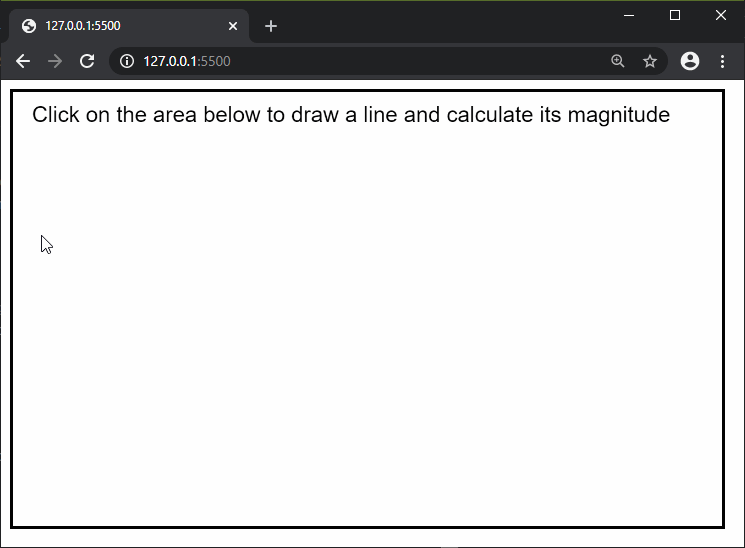GFG App
Open AppBrowser
Continue

The mag() function in p5.js is used to find the magnitude or length of a vector. As a vector does not have a starting position, the magnitude is calculated from position (0, 0) to (x, y). It is equivalent to using dist(0, 0, x, y).

Syntax:

`mag( a, b )`

Parameters: This function accept two parameters as mentioned above and described below:

• a: It is a number which denotes the first value, that is the “x” coordinate of the vector.
• b: It is a number which denotes the second value, that is the “y” coordinate of the vector.

Return Value: It returns a number with the magnitude of the vector.

Below examples illustrate the mag() function in p5.js:

Example:

 `function` `setup() { ` `  ``createCanvas(650, 400); ` `  ``strokeWeight(5); ` `  ``rect(0, 0, width, height); ` `  ``textSize(20); ` ` `  `  ``text(``"Click on the area below to draw"` `          ``+ ``" a line and calculate its "` `          ``+ ``"magnitude"``, 20, 30); ` `} ` ` `  `function` `mousePressed() { ` `  ``strokeWeight(1); ` ` `  `  ``// Draw line to where the ` `  ``// mouse is clicked ` `  ``line(0, 0, mouseX, mouseY); ` ` `  `  ``// Calculate the line magnitude ` `  ``lineMag = mag(mouseX, mouseY); ` ` `  `  ``// Draw the magnitude text on ` `  ``// the end of the line ` `  ``text(lineMag, mouseX, mouseY); ` `} `

Output:Online editor: https://editor.p5js.org/

Reference: https://p5js.org/reference/#/p5/mag

My Personal Notes arrow_drop_up